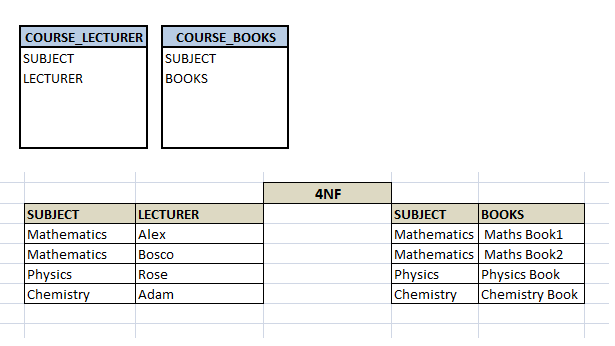Fourth Normal Form (4NF)

Fourth Normal Form (4NF)

In the fourth normal form,

• It should meet all the requirement of 3NF
• Attribute of one or more rows in the table should not result in more than one rows of the same table leading to multi-valued dependencies

To understand it clearly, consider a table with Subject, Lecturer who teaches each subject and recommended Books for each subject.If we observe the data in the table above it satisfies 3NF. But LECTURER and BOOKS are two independent entities here. There is no relationship between Lecturer and Books. In the above example, either Alex or Bosco can teach Mathematics. For Mathematics subject , student can refer either ‘Maths Book1’  or ‘Maths Book2’.  i.e.;

SUBJECT –> LECTURER

SUBJECT–>BOOKS

This is a multivalued dependency on SUBJECT. If we need to select both lecturer and books recommended for any of the subject, it will show up (lecturer, books) combination, which implies lecturer who recommends which book. This is not correct.

SELECT c.LECTURER, c.BOOKS FROM COURSE c WHERE SUBJECT = 'Mathematics';

To eliminate this dependency, we divide the table into two as below:Now if we want to know the lecturer names and books recommended for any of the subject, we will fire two independent queries. Hence it removes the multi-valued dependency and confusion around the data. Thus the table is in 4NF.

--Select the lecturer names
SELECT c.SUBJECT , c.LECTURER  FROM COURSE c WHERE c.SUBJECT = 'Mathematics';

--Select the recommended book names
SELECT c.SUBJECT , c.BOOKS FROM COURSE c WHERE c.SUBJECT = 'Mathematics';